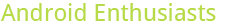## Reputation averages

0

(click on this box to dismiss)Q&A about the site for enthusiasts and power users of the Android operating system

``````DECLARE @exclude_new_users INT
SET @exclude_new_users = ##ExcludeNewUsers:int?0##

DECLARE @excluded_rep_table TABLE (Rep INT)
INSERT INTO @excluded_rep_table VALUES (1),(101)

SELECT COUNT(Id) AS [Total Users],
MIN(Reputation) AS [Minimum],
MAX(Reputation) AS [Maximum],
SUM(Reputation) / COUNT(Id) AS [Mean],
(
SELECT TOP 1 Reputation
FROM Users
WHERE Reputation <>
CASE WHEN @exclude_new_users = 0
THEN -1
ELSE (SELECT IIF (EXISTS (SELECT Rep FROM @excluded_rep_table WHERE Rep = Reputation), Reputation, -1))
END
GROUP BY Reputation
ORDER BY COUNT(Reputation) DESC
) AS [Mode],
(
SELECT
(
(
SELECT MAX(Reputation)
FROM
(
SELECT TOP 50 PERCENT Reputation
FROM Users
WHERE Reputation <>
CASE WHEN @exclude_new_users = 0
THEN -1
ELSE (SELECT IIF (EXISTS (SELECT Rep FROM @excluded_rep_table WHERE Rep = Reputation), Reputation, -1))
END
ORDER BY Reputation
) AS [Bottom Half]
)
+
(
SELECT MIN(Reputation)
FROM
(
SELECT TOP 50 PERCENT Reputation
FROM Users
WHERE Reputation <>
CASE WHEN @exclude_new_users = 0
THEN -1
ELSE (SELECT IIF (EXISTS (SELECT Rep FROM @excluded_rep_table WHERE Rep = Reputation), Reputation, -1))
END
ORDER BY Reputation DESC
) AS [Top Half]
)
) / 2
) AS [Median],
STDEVP(Reputation) AS [Standard Deviation]
FROM Users
WHERE Reputation <>
CASE WHEN @exclude_new_users = 0
THEN -1
ELSE (SELECT IIF (EXISTS (SELECT Rep FROM @excluded_rep_table WHERE Rep = Reputation), Reputation, -1))
END
AND Reputation < 90000``````

### Enter Parameters

Options:
-Hold tight while we fetch your results
:records returned in :time ms:cached